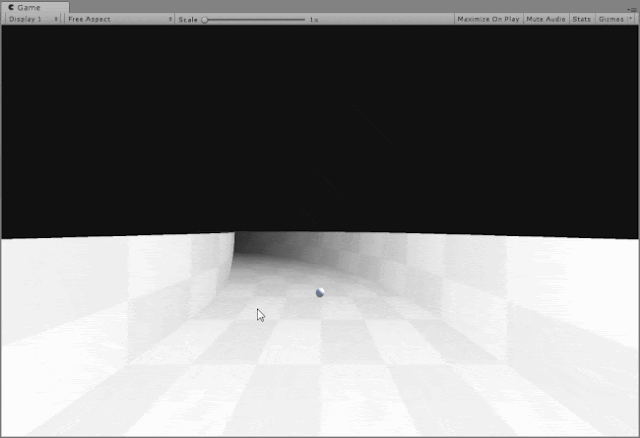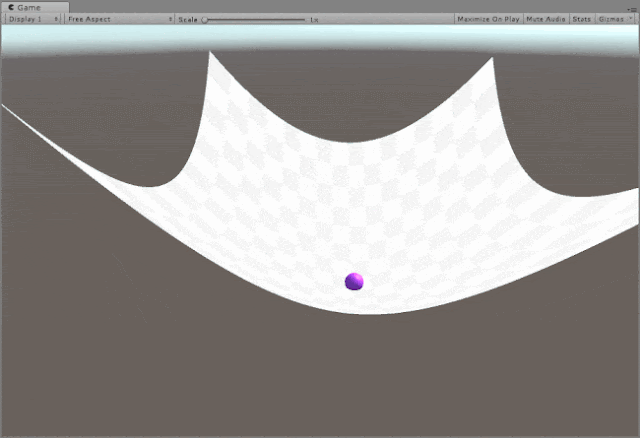### Curved Surface Shader [ Unity Implementation ]

Curved Surface Shader
This is the shader that we will be having at the end of this tutorial.Endless Runner ExampleGravity Visualizer
The curved surface shader is capable of achieving really varied visual effects from showing space-time curve due to gravity to a generic curved world shader that is seen in endless runners like Subway Surfers.
The concepts that you learn here can open you up to a new way of looking at shaders and if you didn't think they were the coolest thing ever already, hopefully let this be the turning point.😝.

Both the examples show above use the same exact material is just that different values have been passed to the shader.
Start by creating a new unlit shader in Unity and we will work our way from there.
First we define what the properties are:
``````_MainTex("Texture", 2D) = "white" {}
_BendAmount("Bend Amount", Vector) = (1,1,1,1)
_BendOrigin("Bend Origin", Vector) = (0,0,0,0)
_BendFallOff("Bend Falloff", float) = 1.0
_BendFallOffStr("Falloff strength", Range(0.00001,10)) = 1.0
``````
This is how they are defined in the CG Program section :
``````float3 _BendAmount;
float3 _BendOrigin;
float _BendFallOff;
float _BendFallOffStr;
``````
Now let's look at the vertex shader:
``````v2f vert(appdata v)
{
v2f o;
o.vertex = UnityObjectToClipPos(curveIt(v.vertex));
o.uv = v.uv;
UNITY_TRANSFER_FOG(o,o.vertex);
return o;
}
``````
As you can we have a function called 'curveIt' which processes the input vertex values and passes it onto to be converted to clip space.
Now the gravy of the entire shader - The CurveIt Function:
``````float4 curveIt(float4 v)
{
/*1*/float4 world = mul(unity_ObjectToWorld, v);
/*2*/float dist = length(world.xyz - _BendOrigin.xyz);
/*3*/dist = max(0, dist - _BendFallOff);

/*4*/dist = pow(dist, _BendFallOffStr);
/*5*/world.xyz += dist * _BendAmount;
/*6*/return mul(unity_WorldToObject, world);
}
``````
We will now go through those 6 lines of code that do all the magic.
1. Getting world space location of a particular vertex and save it in 'world'
2. Calculating the distance between the vertex position and where the _BendOrigin is.
3. dist value is prevented from going below 0, This prevents undefined behaviour when using pow function (-ve values don't work with pow).
4. dist value is raised to _BendFallOffStr value, which defines the steepness of the curve itself.
5. _BendAmount should be dependent on the distance value(dist) so as to have a falloff curve, So we simply multiply it with dist and add to 'world' (position of vertex in world).
6. convert the new world space of vertex and convert back to local( object ) space.
There are no changes to fragment shader in our case.
Now it's just a matter of playing around with the material properties. This shader works best when there are enough vertices to work with. So the default Unity cube is not a good candidate. The Unity plane is a better option but still it's not enough vertices to work with... so multiple Unity planes arranged in a grid with the Curved Surface shader applied on them is the best way to test it out quickly.... or you can make a high poly plane in Blender then import it.

To make the Gravity Displacement example shown above it's just a couple lines of code and attaching that to a sphere primitive.
``````using UnityEngine;
public class CurvedShaderTester : MonoBehaviour
{
[SerializeField] private Material curvedSurfaceMat;
void Start()
{
curvedSurfaceMat.SetVector("_BendAmount", new Vector3(0, 0.01f, 0));
curvedSurfaceMat.SetFloat("_BendFallOff", -9.8f);
curvedSurfaceMat.SetFloat("_BendFallOffStr", 2.0f);
}
void Update()
{
curvedSurfaceMat.SetVector("_BendOrigin", transform.position);
}
}
``````
That's literally it.
To make the Endless Runner example,we just have to modify the _BendAmount by keeping the y-axis bend as constant and changing the value either on the x-axis or z-axis depends on your case. This time instead of a sphere, We attach the script on the Camera itself.
``````using UnityEngine;
public class CurvedShaderTester : MonoBehaviour
{
[SerializeField] private Material curvedSurfaceMat;
void Start()
{
curvedSurfaceMat.SetFloat("_BendFallOff", 17.0f);
curvedSurfaceMat.SetFloat("_BendFallOffStr", 2.0f);
}

void Update()
{
curvedSurfaceMat.SetVector("_BendOrigin", transform.position);
curvedSurfaceMat.SetVector("_BendAmount", new Vector3(0, -0.01f, (Mathf.Sin(Time.time) * 0.03f)));
}
}
``````
Support Bitshift Programmer by leaving a like on Bitshift Programmer Facebook Page and be updated as soon as there is a new blog post.
If you have any questions that you might have about shaders, C# or Unity development in general don't be shy and leave a message on my facebook page or down in the comments.

For more shader goodness, Go HERE.
For Unity game development stuff, Go HERE.